#### Last visited songs

No songs visited yet...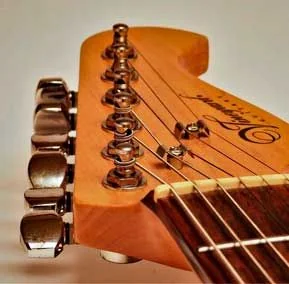# Milk Shakin Momma chords by Dan Hicks## Chords with lyrics

```Milk Shakin' Momma     Dan Hicks    Key ofC majorC   notes below
--[email protected]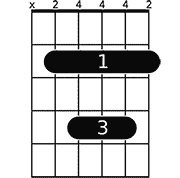CbCbC majorCCbCbC majorC
I sat ..CbCbC majorC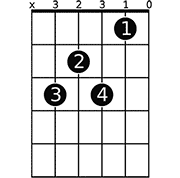C7C7
When I ...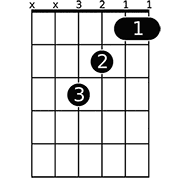FF                 F,E,F
* I ...C majorC                C,Cb,C
Saw ..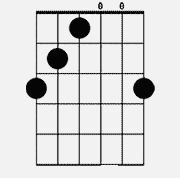G+G                            G,F#,F
I ..C majorC
And ...

She said"..
And ..
->*FF
CH: Raspberry ...C majorC
Even ..FF
Marishino ..C majorC
Bananas ..FF
And ..C majorC
As I ..G+G
She's the ..FFC majorC
And I ..

--Instrumental-----C majorC
Luckily ..C majorC
Later ..

That's ..
She's ..
[end]
c,a7 f,              f#dim   c       a
Next ...
d7           g            c
Try the ..
c7
It ...     etc......            * [email protected]=
.com *

I'm not really sure which diminished chord this is, it looks lik=
e this
x o
=DA=C4=C2=C4=C2=C4=C2=C4=C2=C4=BF      =DA=C4=C43=C4=C4=C4=
=C4=C4    I play theCbCb by simply moving all fingers
=B3 =B3 1 1 1 1      =C3=C4=C41=C4=C4=C4=C4=C4    down one fre=
t.(lift the 1st finger) then
=C3=C4=C5=C4=C5=C4=C5=C4=C5=C4=B4      =C3=C4=C42=C4=C4=C4=
=C4=C4    slide up. Same with the Fb,
=B3 =B3 =B3 2 =B3 3      =C3=C4=C41=C4=C4=C4=C4
=C3=C4=C5=C4=C5=C4=C5=C4=C5=C4=B4      =C3=C4=C4=C4=C4=C4=C4=
=C4=C4    If you have problems understanding this,
=B3 =B3 =B3 =B3 =B3 =B3      =C0=C4=C4=C4=C4=C4=C4=C4=C4    pl=
ease let me know how I can do it better.
I barre the first fret```

### Welcome to the guitar community

- Get songbook with favourite songs
- Get custom made front page
- Submit chords and tabs
- Submit guitar-cover videos
- Get a lot of thumbs up for your work

#### Last visited songs

No songs visited yet...

Site
Home
Search
Community
Submit chords
Submit video

### Video

No video for this page. Check out how you can submit guitar-cover videos here